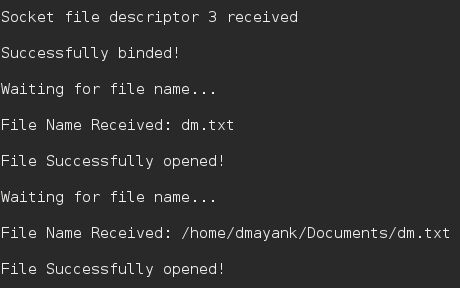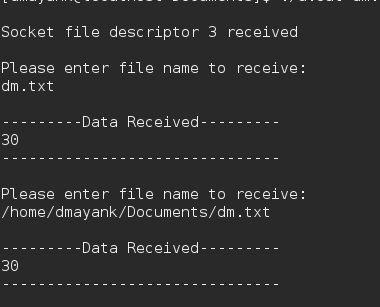Open in App
Not now

# C program for file Transfer using UDP

• Difficulty Level : Medium
• Last Updated : 30 Jul, 2019

Data can be transferred between two computers using Socket programming in C.

Similarly, files can easily be sent using UDP protocol and a simple client/server.

Security: Handled by encryption.

Protocol : UDP
Encryption: XOR encryption

Algorithm :

```1. The server starts and waits for filename.
2. The client sends a filename.
If file is present,
and continues to send a buffer filled with
file contents encrypted until file-end is reached.
4. End is marked by EOF.
5. File is received as buffers until EOF is
```

The Server :

 `// server code for UDP socket programming ` `#include ` `#include ` `#include ` `#include ` `#include ` `#include ` `#include ` `#include ` ` `  `#define IP_PROTOCOL 0 ` `#define PORT_NO 15050 ` `#define NET_BUF_SIZE 32 ` `#define cipherKey 'S' ` `#define sendrecvflag 0 ` `#define nofile "File Not Found!" ` ` `  `// function to clear buffer ` `void` `clearBuf(``char``* b) ` `{ ` `    ``int` `i; ` `    ``for` `(i = 0; i < NET_BUF_SIZE; i++) ` `        ``b[i] = ``'\0'``; ` `} ` ` `  `// function to encrypt ` `char` `Cipher(``char` `ch) ` `{ ` `    ``return` `ch ^ cipherKey; ` `} ` ` `  `// function sending file ` `int` `sendFile(``FILE``* fp, ``char``* buf, ``int` `s) ` `{ ` `    ``int` `i, len; ` `    ``if` `(fp == NULL) { ` `        ``strcpy``(buf, nofile); ` `        ``len = ``strlen``(nofile); ` `        ``buf[len] = EOF; ` `        ``for` `(i = 0; i <= len; i++) ` `            ``buf[i] = Cipher(buf[i]); ` `        ``return` `1; ` `    ``} ` ` `  `    ``char` `ch, ch2; ` `    ``for` `(i = 0; i < s; i++) { ` `        ``ch = ``fgetc``(fp); ` `        ``ch2 = Cipher(ch); ` `        ``buf[i] = ch2; ` `        ``if` `(ch == EOF) ` `            ``return` `1; ` `    ``} ` `    ``return` `0; ` `} ` ` `  `// driver code ` `int` `main() ` `{ ` `    ``int` `sockfd, nBytes; ` `    ``struct` `sockaddr_in addr_con; ` `    ``int` `addrlen = ``sizeof``(addr_con); ` `    ``addr_con.sin_family = AF_INET; ` `    ``addr_con.sin_port = htons(PORT_NO); ` `    ``addr_con.sin_addr.s_addr = INADDR_ANY; ` `    ``char` `net_buf[NET_BUF_SIZE]; ` `    ``FILE``* fp; ` ` `  `    ``// socket() ` `    ``sockfd = socket(AF_INET, SOCK_DGRAM, IP_PROTOCOL); ` ` `  `    ``if` `(sockfd < 0) ` `        ``printf``(``"\nfile descriptor not received!!\n"``); ` `    ``else` `        ``printf``(``"\nfile descriptor %d received\n"``, sockfd); ` ` `  `    ``// bind() ` `    ``if` `(bind(sockfd, (``struct` `sockaddr*)&addr_con, ``sizeof``(addr_con)) == 0) ` `        ``printf``(``"\nSuccessfully binded!\n"``); ` `    ``else` `        ``printf``(``"\nBinding Failed!\n"``); ` ` `  `    ``while` `(1) { ` `        ``printf``(``"\nWaiting for file name...\n"``); ` ` `  `        ``// receive file name ` `        ``clearBuf(net_buf); ` ` `  `        ``nBytes = recvfrom(sockfd, net_buf, ` `                          ``NET_BUF_SIZE, sendrecvflag, ` `                          ``(``struct` `sockaddr*)&addr_con, &addrlen); ` ` `  `        ``fp = ``fopen``(net_buf, ``"r"``); ` `        ``printf``(``"\nFile Name Received: %s\n"``, net_buf); ` `        ``if` `(fp == NULL) ` `            ``printf``(``"\nFile open failed!\n"``); ` `        ``else` `            ``printf``(``"\nFile Successfully opened!\n"``); ` ` `  `        ``while` `(1) { ` ` `  `            ``// process ` `            ``if` `(sendFile(fp, net_buf, NET_BUF_SIZE)) { ` `                ``sendto(sockfd, net_buf, NET_BUF_SIZE, ` `                       ``sendrecvflag,  ` `                    ``(``struct` `sockaddr*)&addr_con, addrlen); ` `                ``break``; ` `            ``} ` ` `  `            ``// send ` `            ``sendto(sockfd, net_buf, NET_BUF_SIZE, ` `                   ``sendrecvflag, ` `                ``(``struct` `sockaddr*)&addr_con, addrlen); ` `            ``clearBuf(net_buf); ` `        ``} ` `        ``if` `(fp != NULL) ` `            ``fclose``(fp); ` `    ``} ` `    ``return` `0; ` `} `

The Client:

 `// client code for UDP socket programming ` `#include ` `#include ` `#include ` `#include ` `#include ` `#include ` `#include ` `#include ` ` `  `#define IP_PROTOCOL 0 ` `#define IP_ADDRESS "127.0.0.1" // localhost ` `#define PORT_NO 15050 ` `#define NET_BUF_SIZE 32 ` `#define cipherKey 'S' ` `#define sendrecvflag 0 ` ` `  `// function to clear buffer ` `void` `clearBuf(``char``* b) ` `{ ` `    ``int` `i; ` `    ``for` `(i = 0; i < NET_BUF_SIZE; i++) ` `        ``b[i] = ``'\0'``; ` `} ` ` `  `// function for decryption ` `char` `Cipher(``char` `ch) ` `{ ` `    ``return` `ch ^ cipherKey; ` `} ` ` `  `// function to receive file ` `int` `recvFile(``char``* buf, ``int` `s) ` `{ ` `    ``int` `i; ` `    ``char` `ch; ` `    ``for` `(i = 0; i < s; i++) { ` `        ``ch = buf[i]; ` `        ``ch = Cipher(ch); ` `        ``if` `(ch == EOF) ` `            ``return` `1; ` `        ``else` `            ``printf``(``"%c"``, ch); ` `    ``} ` `    ``return` `0; ` `} ` ` `  `// driver code ` `int` `main() ` `{ ` `    ``int` `sockfd, nBytes; ` `    ``struct` `sockaddr_in addr_con; ` `    ``int` `addrlen = ``sizeof``(addr_con); ` `    ``addr_con.sin_family = AF_INET; ` `    ``addr_con.sin_port = htons(PORT_NO); ` `    ``addr_con.sin_addr.s_addr = inet_addr(IP_ADDRESS); ` `    ``char` `net_buf[NET_BUF_SIZE]; ` `    ``FILE``* fp; ` ` `  `    ``// socket() ` `    ``sockfd = socket(AF_INET, SOCK_DGRAM, ` `                    ``IP_PROTOCOL); ` ` `  `    ``if` `(sockfd < 0) ` `        ``printf``(``"\nfile descriptor not received!!\n"``); ` `    ``else` `        ``printf``(``"\nfile descriptor %d received\n"``, sockfd); ` ` `  `    ``while` `(1) { ` `        ``printf``(``"\nPlease enter file name to receive:\n"``); ` `        ``scanf``(``"%s"``, net_buf); ` `        ``sendto(sockfd, net_buf, NET_BUF_SIZE, ` `               ``sendrecvflag, (``struct` `sockaddr*)&addr_con, ` `               ``addrlen); ` ` `  `        ``printf``(``"\n---------Data Received---------\n"``); ` ` `  `        ``while` `(1) { ` `            ``// receive ` `            ``clearBuf(net_buf); ` `            ``nBytes = recvfrom(sockfd, net_buf, NET_BUF_SIZE, ` `                              ``sendrecvflag, (``struct` `sockaddr*)&addr_con, ` `                              ``&addrlen); ` ` `  `            ``// process ` `            ``if` `(recvFile(net_buf, NET_BUF_SIZE)) { ` `                ``break``; ` `            ``} ` `        ``} ` `        ``printf``(``"\n-------------------------------\n"``); ` `    ``} ` `    ``return` `0; ` `} `

Output :
Server :Client :My Personal Notes arrow_drop_up
Related Articles